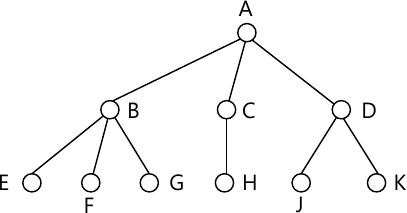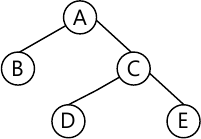MORE IN Data Structures Algorithm
SPPU Computer Engineering (Semester 3)
Data Structures Algorithm
December 2013
Total marks: --
Total time: --
INSTRUCTIONS
(1) Assume appropriate data and state your reasons
(2) Marks are given to the right of every question
(3) Draw neat diagrams wherever necessary

Answer any one question from Q1 and Q2
1 (a) Draw the IPO chart for obtaining the solution for 'Calculating the area of Triangle'.
3 M
1 (b) Define the terms with respect to sorting: internal sort, external sort and sorting stability.
3 M
1 (c) Convert the following generalized tree into a binary tree.3 M
1 (d) What is the necessity of threaded binary tree? Define the terms inorder successor and inorder predecessor with respect to inorder threaded binary tree.
3 M

2 (a) Find the frequency count of the following code:
for(i=0;i {
for(j=0;j {
c[i][j] = 0;
for(k=0;k c[i][j] = a[i][k] × b[k][j];
}
}
3 M
2 (c) Represent the following binary tree using array.3 M
2 (d) Write a pseudo C/C++ code for any of the recursive depth first traversal of the binary tree.
3 M
2(b) Sort the following data in ascending order using Radix Sort: 25, 06, 45, 60, 140, 50.
3 M

Answer any one question from Q3 and Q4
3 (a) Explain any three applications of graphs in the area of Computer Engineering.
3 M
3 (b) Differentiate between Prim's and Kruskal's algorithm for generating the spanning tree of the graph.
3 M
3 (c) Create an AVL tree for the following data by inserting it in an order one at a time:
10, 20, 15, 12, 25, 30.
4 M
3 (d) Enlist the names of static tree tables with suitable example.
2 M

4 (a) Explain the situation in which linked representation of a graph is more beneficial than array representation.
2 M
4 (b) Write a pseudo C/C++ code for finding depth first traversal of the graph.
4 M
4 (c) What is the use of hash tables? Enlist the characteristics of good hash function.
3 M
4 (d) Assume the size of hash table as 8. The hash function to be used to calculate the hash value of the data X is: X%8. Insert the following values in hash table: 10, 12, 20, 18, 15. Use linear probing without replacement for handling collision.
3 M

Answer any one question from Q5 and Q6
5 (a) Write a pseudo C/C++ code to sort the data in ascending order using heap sort.
6 M
5 (b) Create a B tree of order 3 for the following data : 20, 10, 30, 15, 12, 40, 50.
4 M
5 (c) Explain the various modes of opening the file in C or C++.
3 M

6 (a) Sort the following data in ascending order using heap sort: 15, 10, 40, 25.
4 M
6 (b) Write a pseudo C/C++ code to search the data stored in a B tree.
6 M
6 (c) Explain any three operations carried out on sequential files.
3 M

Answer any one question from Q7 and Q8
7 (a) Write a parallel algorithm to calculate the addition of numbers stored in an array using prefix computation method.
6 M
7 (b) Explain the various models used for parallel computation.
4 M
7 (c) Explain pointer doubling problem in brief.
3 M

8 (a) Write a parallel algorithm for odd - even merge sort. Explain the algorithm with suitable Example.
7 M
8 (b) Write a parallel algorithm to perform the addition of the given numbers using complete binary tree method.
6 M

More question papers from Data Structures Algorithm